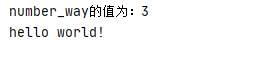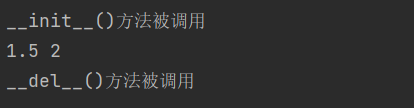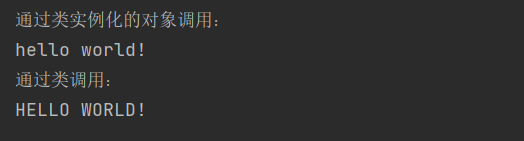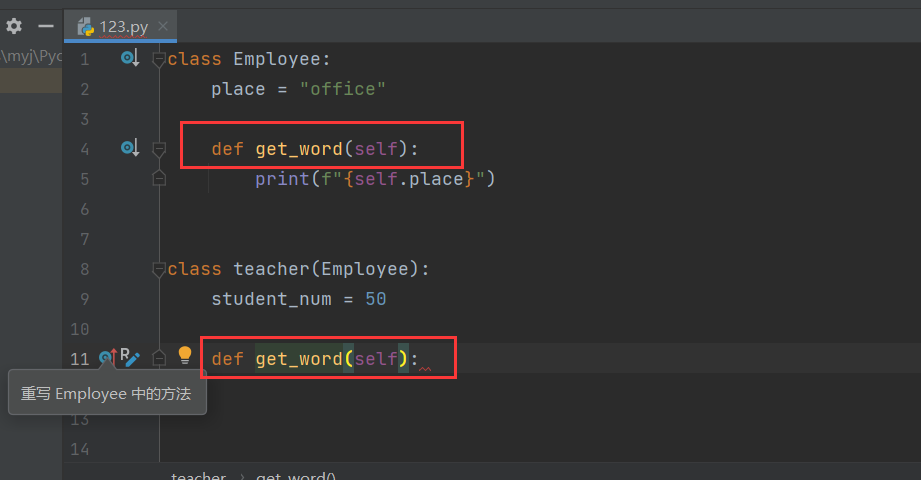# 一、类

## （一）类、对象

Python是一种面向对象语言，面向对象有两个概念，分别是类和对象。

`对象是类的实例`，用于描述现实中的个体。

## （二）类的定义

``````class 类的名称
属性名称=属性值
def 方法名称(self):    #self是一个指向对象的默认参数，类中必须包含参数 self, 且为第一个参数。
方法体
``````

``````class Way:
number_way = 3

def world(self):
print("hello world!")

``````

## （三）对象的创建和使用

``````对象名称=类名称()
``````

``````对象名称.属性

``````

``````class Way:
number_way = 3

def world(self):
print("hello world!")

a = Way()
print(a.number_way)
a.world()

``````# 二、限制对象访问

## （一）定义私有成员、方法

``````class Way:
__number_way = 3

def __world(self):
print("hello world!")

``````

## （二）访问私有成员、方法

``````class Way:
__number_way = 3

def __world(self):  # 私有方法
print("hello world!")

def get_way(self):  # 公有方法
print(f"number_way的值为：{

self.__number_way}")  # 私有成员通过公有方法中的指代类本身的默认参数self访问
self.__world()  # 私有方法通过公有方法中的指代类本身的默认参数self访问

a = Way()
a.get_way()

``````# 三、构造方法和析构方法

## （一）构造方法

``````class Triangle:
def __init__(self, bottom, high):
self.bottom = bottom
self.high = high

def get_s(self):
s = (self.bottom * self.high) / 2.0
print(f"三角形的面积为：{

s}")

S = Triangle(1.5, 2)
print(S.bottom, S.high)
S.get_s()

``````## （二）析构方法

``````class Triangle:
def __init__(self, bottom, high):
self.bottom = bottom
self.high = high
print("__init__()方法被调用")

def __del__(self):
print("__del__()方法被调用")

def get_s(self):
s = (self.bottom * self.high) / 2.0
print(f"三角形的面积为：{

s}")

S = Triangle(1.5, 2)
print(S.bottom, S.high)

``````# 四、类方法和静态方法

## （一）类方法

``````class GetW:
word = "hello world!"

@classmethod
def print_word(cls):
print(f"{

cls.word}")
cls.word = "HELLO WORLD!"  # 类方法修改类属性的值为HELLO WORLD!

A = GetW()  # 创建一个GetW类的对象A
print("通过类实例化的对象调用：")
A.print_word()
print("通过类调用：")
GetW.print_word()

``````## （二）静态方法

``````class Get:
number = 0

@staticmethod
def print_number():
print(f"{

Get.number}")

A = Get()
A.print_number()
Get.print_number()

``````# 五、继承

``````class 派生类名称(基类名称):
``````

``````class Employee:
place = "office"

def get_word(self):
print(f"{

self.place}")

class Teacher(Employee):  # 继承Employee类
student_num = 50  # 子类自己定义的属性

def get_word_num(self):  # 子类自己定义的方法
print(f"教师办公地点为{

self.place}")
print(f"学生人数为{

self.student_num}")

A = Teacher()
print(Teacher.place)  # 子类继承了父类的属性，所以子类有父类的属性
A.get_word()  # 子类继承了父类的方法，所以子类有父类的方法
print(Teacher.student_num)  # 输出子类自己定义的属性
A.get_word_num()  # 调用子类自己定义的方法

``````## （二）派生类方法重写``````class Employee:
place = "office"

@classmethod
def get_word(cls):
print(f"{

cls.place}")

class Teacher(Employee):
student_num = 50

def get_word(self):
print(f"{

self.student_num}")

A = Teacher()
Employee.get_word()
A.get_word()
Employee.get_word()

``````## （三）super()函数

``````class Employee:
place = "office"

@classmethod
def get_word(cls):
print(f"{

cls.place}")

class Teacher(Employee):
student_num = 50

def get_word(self):
print(f"{

self.student_num}")
print("使用super()函数调用基类中的方法：")
super().get_word()

A = Teacher()
A.get_word()

``````# 六、多态

``````class Person:
def get_word(self, name):
name.get_word()

class Employee:
def get_word(self):
print("职工")

class Teacher(Employee):
def get_word(self):
print("教师")

class Professor(Employee):
def get_word(self):
print("教授")

A = Person()
A.get_word(Employee())
A.get_word(Teacher())
A.get_word(Professor())

``````